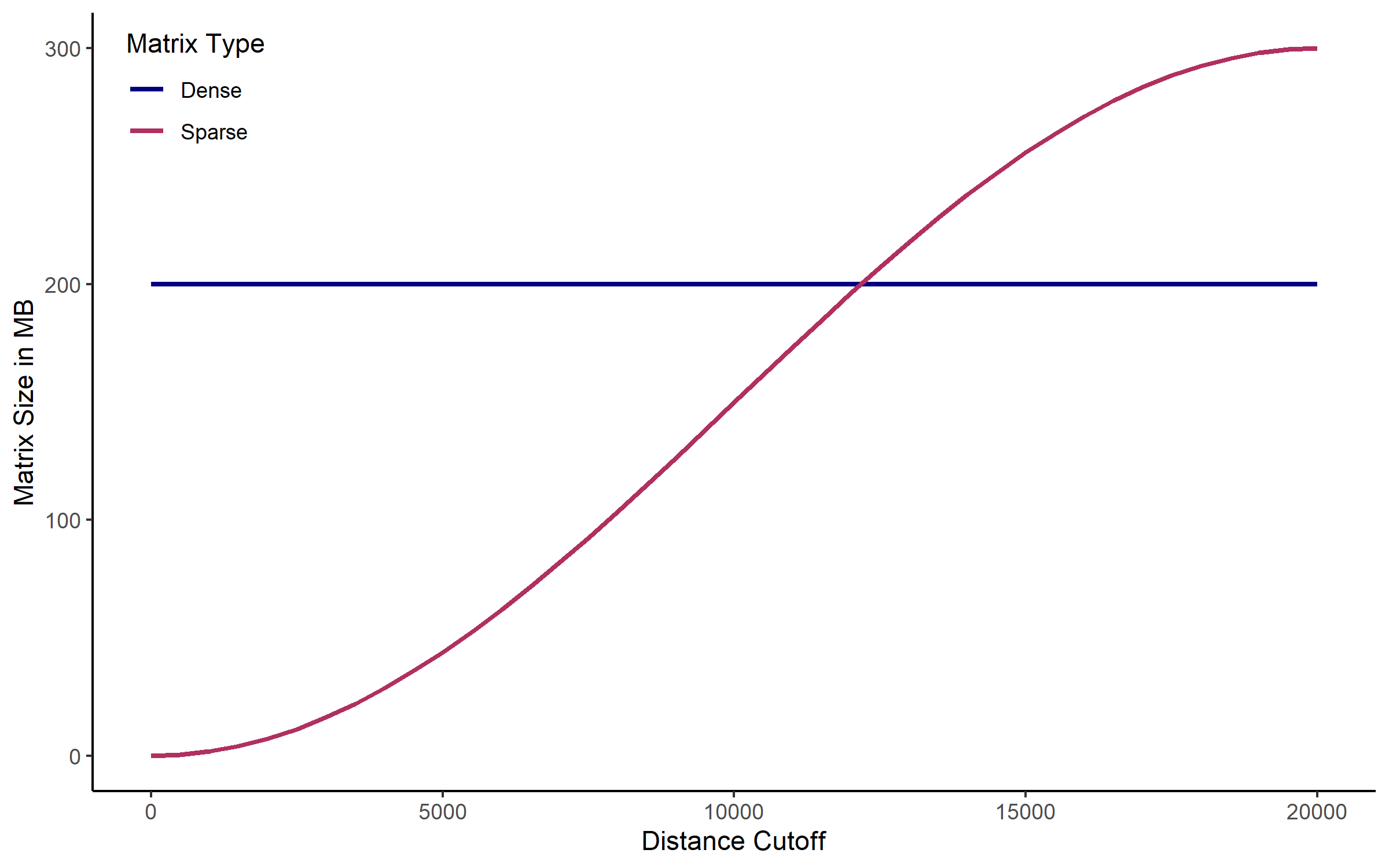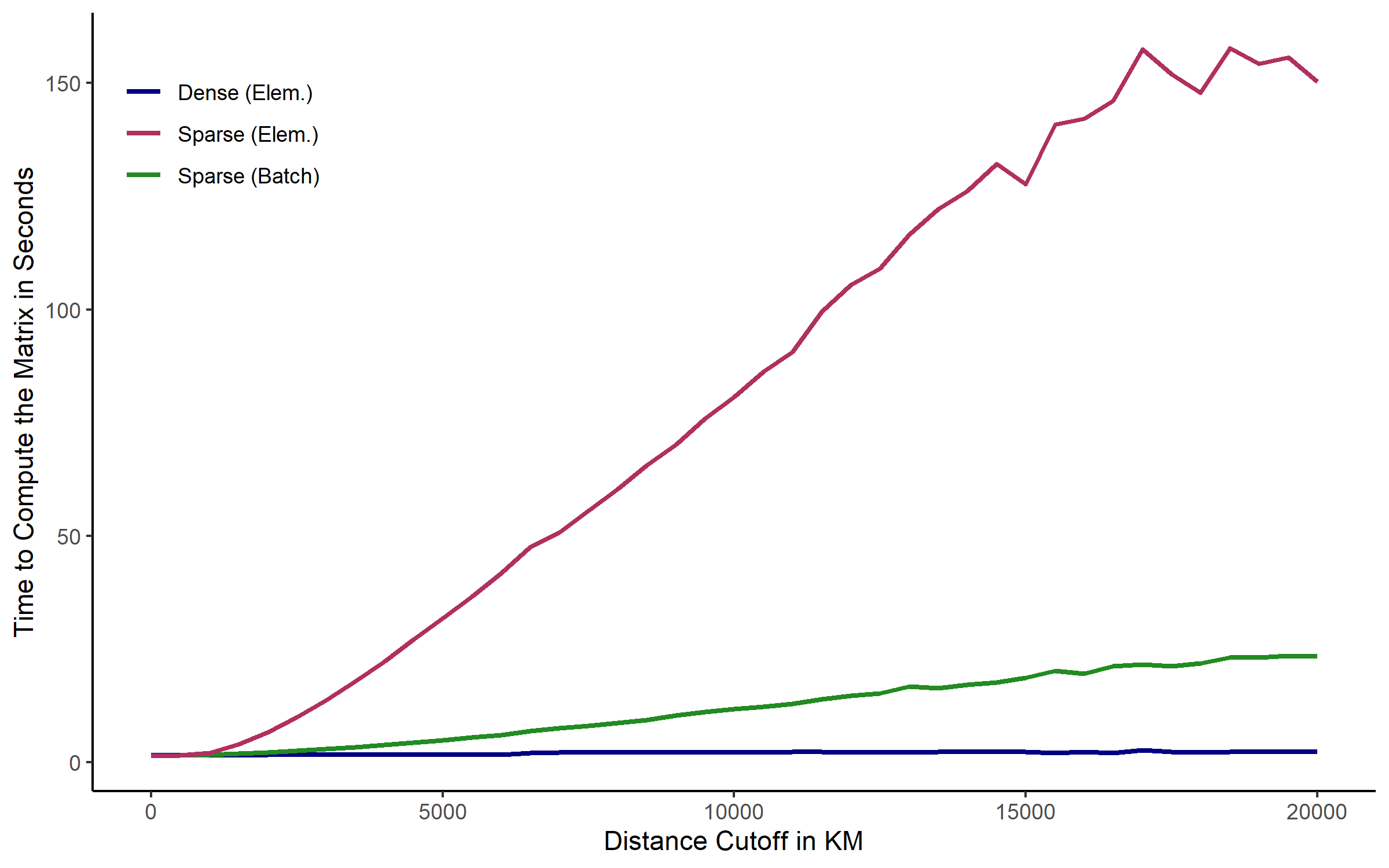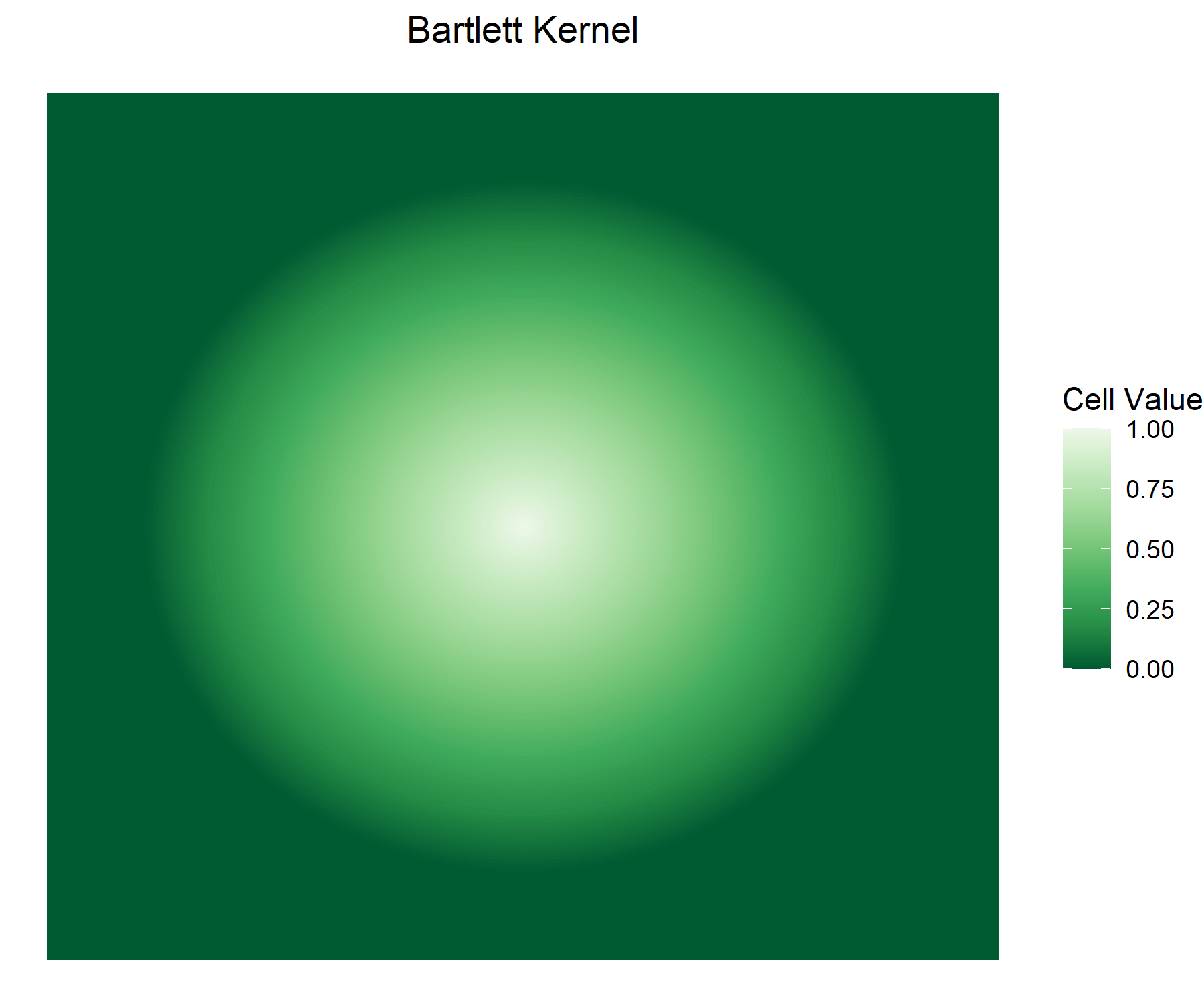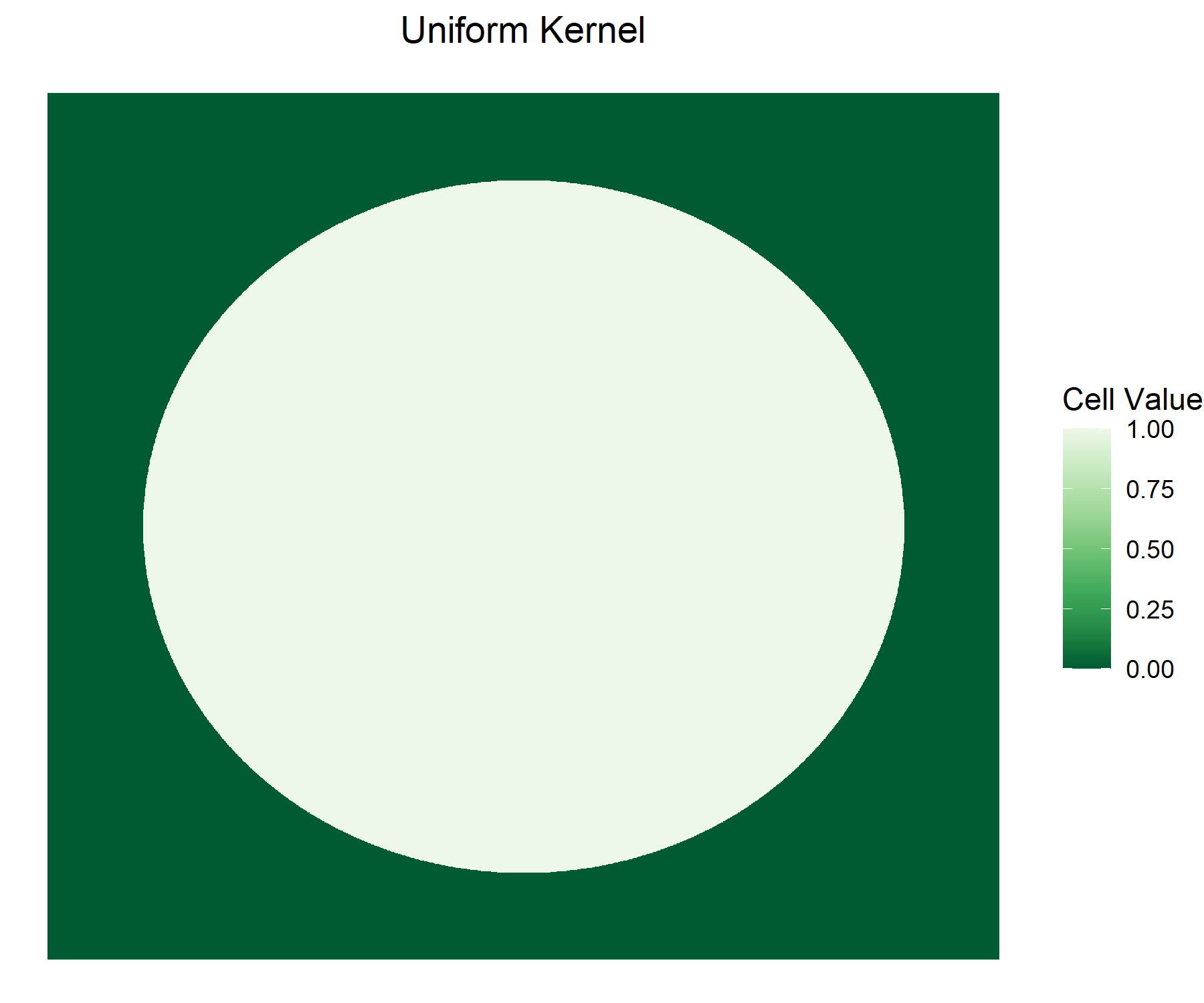# 1 Introduction

Conley (1999, 2008) standard errors account for spatial correlation in the data. Just like clustered standard errors consider observations not be independent of each other within groups, Conley standard errors recognize potential dependence based on spatial proximity. As with other common standard error corrections, Conley standard errors do not require adjustments to the overall estimation strategy. They can be applied to an otherwise non-spatial model, such as OLS. The coefficient estimates remain untouched and non-spatial in that case and only the standard errors are adjusted.

Since Conley (1999) published his paper and Stata code on the proposed method, various other software implementations have been released, each building on earlier versions. Gradual modifications and extensions led to a growing number of functionalities and computational optimization over time. Among these earlier implementations are e.g. scripts and packages by (i) Richard Bluhm, (ii) Luis Calderon and Leander Heldring, (iii) Darin Christensen and Thiemo Fetzer, (iv) Fabrizio Colella, Rafael Lalive, Seyhun Orcan Sakalli, and Mathias Thoenig, and (v) Solomon Hsiang. The conleyreg package is the latest iteration of that process. With its extensive computational optimization, users can efficiently compute Conley standard errors on large data sets, even if it involves internal matrices with billions of cells.

# 2 Distances

All of the above mentioned implementations employ the original econometric method that Conley introduced more than two decades ago. And at least with OLS as accompanying estimation strategy, there is no stochastic component to this standard error correction. Nonetheless, results differ across implementations. The root of these discrepancies is the heterogeneity in distance estimations. Computing spatial distances between observations is key to deriving Conley standard errors, and implementations vary in multiple aspects of this step. The disparities range from a slightly different Earth radius over alternative distance functions to unaligned definitions of space itself. Timothy Conley, Luis Calderon, and Leander Heldring do not restrict their functions to geographic space on Earth, but use a more abstract definition of space with an arbitrary number of dimensions and distance cutoffs delineating intervals along them. The flexibility of this abstract definition allows for a broader variety of applications, but comes with a number of drawbacks when applied to geographic space on Earth. It is somewhat restricted to planar projections, draws distance buffers in two-dimensional space as a rectangular box rather than a circle and faces a series of distortions and measurement errors arising from the planet’s spherical nature. The other packages and scripts focus on geographic distances on Earth, and largely rely on the Haversine equation. Haversine distances are not accurate down to the meter because the planet is not a perfect sphere. However, they are widely adopted in modern GIS software and are faster than more complex alternatives. Google’s S2 library, which the sf package now uses as default, employs that function - and conleyreg does so as well. The Haversine distance function handles spherical geometry and thereby does not apply to projected data. Unlike unprojected data, also referred to as longlat, projected data does not model locations as points on a sphere. It projects them to another spatial representation, usually onto a two-dimensional plain. Such transformation necessarily distorts the data in some regard. Hence, there are many different projections targeting specific purposes. A Mollweide equal-area projection generates equally large pixels. An azimuthal equidistant projection preserves the correct distances between the centroid and other points. Like the sf package, conleyreg uses Euclidean distances for projected data. Those computations do not correct for distortions introduced by the projection or the fact that the Earth is round and it is possible to leave a world map on one side and enter it on the other side. The latter would i.a. require calculating the optimal transition point for any projection, including the non-rectangular ones. Even though Haversine distances on longlat data are not accurate down to the meter, measurement error in distances computed from projected data can be much larger. Hence, it is recommended to use longlat data in conleyreg, unless you are certain that the selected projection gets the distances right.

By default, conleyreg uses Haversine distances on longlat input data and Euclidean distances on projected input data. You can change this behavior by specifying dist_comp = "spherical" or dist_comp = "planar", which refer to Haversine and Euclidean distances respectively. The functions then transform the data before computing distances. The resulting distance values are identical to those produced by sf::st_distance, unless you use sf’s old default (sf::sf_use_s2(FALSE)). In that case, sf employs the GEOS planar library. The difference between conleyreg’s internal distance functions and sf::st_distance is that the former are computationally highly optimized, making use of parallilization and the fact that only values below dist_cutoff have to be stored. You can still make conleyreg use the sf implementation via st_distance = TRUE. Though because of the lower efficiency, this only makes sense in specific applications - e.g. when you insist on using GEOS.

This package is built on memory efficiency, speed, and flexibility regarding applications and user preferences. Deriving distance matrices is the most time consuming task in calculating Conley standard errors. With a small data set of 5,000 observations, there are 25 million bilateral distances linking each point to all other points and itself. With a data set of 50,000 observations, the distance matrix contains 2.5 billion cells. conleyreg is highly optimized to run computations on even larger data at high speeds and efficient RAM usage. A considerable share of the internal code is written in C++, which is not just faster than R, but also allows to use more efficient data types than the ones available in R. While the exported functions conleyreg and dist_mat come with reasonable defaults, they offer a number of parameters with which you can tailor their behavior to your application. The subsequent paragraphs guide you through the options and explain what they imply in more detail.

## 2.1 Sparse Matrices

One feature that you can explicitly control in conleyreg is the use of sparse matrices. As the size of the distance matrix grows exponentially in the number of observations, it quickly surpasses your computer’s memory limit. A data set of 50,000 points implies 2.5 billion matrix cells. This is where sparse matrices come in handy. Instead of storing all cell values, they only store those that are not zero. Unless multiple points share a location, just $$1/N$$ of all cells in a distance matrix - those along the diagonal - are zero. However, conleyreg does not directly store distances in those cells. The function transforms distances into weights based on the specified distance cutoff and kernel. Only cells with a distance below dist_cutoff are assigned a non-zero weight. If this radius is much smaller than the overall sample region, a vast majority of cells is set to zero - assuming an even distribution of points. In that case, a sparse matrix requires less RAM than a conventional dense matrix. Note that this advantage only holds with many zeros. Otherwise, sparse matrices can even be larger than their dense counterparts. We can illustrate the size differences using a global data set of 5,000 points combined with various distance cutoffs and the below described Bartlett kernel:Dense and Sparse Matrix Sizes

The higher the distance cutoff is, the larger the sparse matrix becomes. At 15,000 km it is already substantially larger than the dense counterpart. This is admittedly not exactly what it looks like in conleyreg, as the package keeps the matrix by default in C++, while this plot compares dense and sparse matrices when exported to R. However, the general message still holds. Because sparse matrices are slower to manipulate than their dense counterpart and only offer a size advantage, if many distances are larger than dist_cutoff, conleyreg uses dense matrices by default. Specify sparse = TRUE to use sparse matrices instead.

Because sparse matrices’ compressed format with column-major ordering makes it slow to enter cell values one by one, conleyreg uses batch insertion by default when sparse = TRUE. With batch insertion, we loop through the $$N(N-1)/2$$ unique bilateral distances (the matrix is symmetric - point A is as far from point B as point B is from A - and values along the diagonal - the distance between a point and itself - are zero), as we would do otherwise, but do not directly insert the values into the sparse matrix. Instead, we store the values and their indices in intermediate dense objects and then batch insert them into the sparse matrix all at once. That requires more RAM, but is much faster than direct insertion. Specify batch = FALSE to use direct insertion instead.

In the case of batch insertion, conleyreg allows to further play around with the trade-off between RAM efficiency and speed. The batch_ram_opt parameter controls the removal of the batch insertion’s intermediate objects. Permitted values are "none", "moderate" (default), and "heavy", where RAM efficiency increases and speed decreases from "none" to "heavy".Speed of Element-Wise and Batch Insertion

The plot compares the computational speed of the three above described settings: element-wise insertion into a dense matrix, element-wise insertion into a sparse matrix, and batch insertion into a sparse matrix. In this case, the functions compute Haversine distances and convert them into weights based on the Bartlett kernel. Each derived matrix contains 100 million cells. Batch insertion uses batch_ram_opt = "moderate". All three functions are parallelized across eight CPU cores, but differ largely in their computational time. While the dense matrix function takes on average around two seconds to compute the 100 million cell matrix, the element-wise sparse matrix alternative takes up to 2.5 minutes to complete the exercise. If you choose to use sparse matrices nonetheless, it is adviseable to try batch insertion before utilizing the element-wise approach.

## 2.2 Data Types

When using conleyreg’s internal distance computations, i.e. st_distance = FALSE, it keeps the distance matrix or weights matrix in C++. Accordingly, the function can make use of data types that do not exist in R. With kernel = "uniform", the function stores values as short integers, each of which is two bytes in size. R, in contrast, only knows regular integers, measuring four bytes each. With kernel = "bartlett", you can choose between doubles (float = FALSE) and floats (float = TRUE). The former occupies eight bytes per value, as it would do in R, whereas the latter occupies four bytes per value. However, because floats store numbers at lower precision than doubles do, this choice can affect the results. In conclusion, you should only use floats, if your machine is unable to accomodate both a dense (sparse = FALSE) and a sparse matrix (sparse = TRUE). The above mentioned 2.5 billion matrix cells, thus, occupy 20GB when using doubles, 10GB when using floats, and 5GB when using short integers in a dense matrix. Sparse matrices can use the same data types, but the overall matrix size depends on how many values are below dist_cutoff.

Whereas conleyreg directly stores the weights, without storing the distances they are based on, the dist_mat function does return the actual distance values. And because these are exported to R, the float data type is not available. By specifying dist_round = TRUE, you can, however, round the distances to full kilometers. The resulting cell values then occupy four instead of eight bytes each.

## 2.3 Row-Wise Distances

As the above paragraphs illustrate, this package offers a number of options with which you can control distance matrices’ RAM requirements. And irrespective of whether you use sparse matrices, the float data type, or rounded distances, the functions loop over $$N(N-1)/2$$ unique bilateral distances, given symmetry and zeros along the diagonal. The difference between $$N(N-1)/2$$ and $$N^2$$ can be billions of iterations in the case of larger data sets. Making use of this speed advantage implies computing the full distance matrix before inserting its values into the standard error corrections.

Dropping the $$N(N-1)/2$$ technique, we can avoid storing the entire distance matrix. The spatial sandwich derivations do not make use of the full distance matrix at once anyway, but loop over its rows. By specifying rowwise = TRUE, you instruct the function to directly insert rows into the subsequent computations. The function then only has to store $$N \times$$ ncores distance matrix cells at once. Though, because it discards rows after use, it has to derive $$N(N-1)$$ distances - twice as many as in the default rowwise = FALSE application.

The rowwise parameter is one out of various options in conleyreg targeting a trade-off between computational speed and RAM efficiency. It can e.g. be useful when the machine’s RAM space is insufficient but the dist_cutoff is too high for sparse matrices to offer a size advantage.

## 2.4 Pre-Computed Distances

When estimating a model through conleyreg, it is usually the computationally best solution to let the function derive distances internally. Yet, there are a few cases in which you might want to pre-compute distance matrices. One example is an application where you estimate a number of models on the exact same data, e.g. trying a number of different distance cutoffs. Then, it is inefficient to have the function re-estimate the same distances in each specification. Instead, you can compute distances once and then pass them to conleyreg via the dist_mat parameter. While you can enter distances derived through functions outside this package, using conleyreg’s dist_mat function is the recommended way. It does not only make sure that the distances are stored in the right format, but is also computationally optimized and pre-computes other results that are constant across estimations on the same data. Employing functions outside the conleyreg package is mostly appropriate when requiring a distance function that the package does not include - e.g. Tobler’s hiking function, which is based on topography and expresses distances in terms of travel time.

In general, you must not modify the input data between the distance estimation and running conleyreg. That includes dropping observations or changing values of the unit, time, or coordinate variables. In cross-sectional settings, you must not re-order rows either. If you compute distances through a function other than dist_mat, there are a few additional issues to account for. (i) In the panel scenario, you must order observations by time and unit in ascending order. I.e. cells [1, 2] and [2, 1] of the distance matrix must refer to the distance between unit 1 and unit 2, cells [2, 3] and [3, 2] to the distance between unit 2 and unit 3 etc. The unit numbers in this example refer to their rank when they are sorted. (ii) dist_cutoff does not refer to kilometers, but to the units of the matrix. (iii) While in a balanced panel you only enter one matrix that is applied to all periods, you supply distances as a list of matrices in the unbalanced case. The matrices must be sorted, with the first list element containing the first period’s distance matrix etc. (iv) Zeros in sparse matrices are interpreted as values above the distance cutoff and NaN values are interpreted as zeros. (v) The matrices in the list must all be of the same type - all dense or all sparse. (vi) Distance matrices must only contain non-missing, finite numbers (and NaN in the case of sparse matrices).

Because the dist_mat function exports distances to R and does not keep them in C++, the float data type is not available. To reduce RAM requirements nonetheless, dist_mat offers a rounding option. With dist_round = TRUE, it rounds distances to full kilometers, storing them in a four byte integer rather than the default eight byte numeric format. Another way to reduce the output object’s size is to set a dist_cutoff combined with sparse = TRUE. Though note that the dist_cutoff used in subsequent conleyreg calls must not be larger than the dist_cutoff set in the dist_mat function.

# 3 Kernels

As discussed in the above section, the computationally costly part of conleyreg is setting up and modifying an N x N matrix that is based on bilateral distances. Because we do not actually enter the distances into that matrix, but transform them first, we can benefit from sparse matrices. Distances above dist_cutoff are set to zero and those below are modified according to one out of two kernels. You can choose between a Bartlett kernel and a uniform kernel. The values they generate are essentially weights with which pixels within the specified radius enter subsequent calculations. With a Bartlett kernel, the weights gradually diminish with distance. With a uniform kernel, all points within the radius are assigned an equal weight of one. The subsequent plots illustrate these weights at given distances from a centrally located point:Weights According to Different Kernels

The Bartlett kernel is the default because it usually makes sense to assign a higher weight to nearer points than to points that are farther away. The uniform kernel does not implement a gradual transition but provides a computational advantage: it only produces zeros and ones. The respective C++ code stores them as short integers which only require at two bytes a quarter of the RAM space that the eight bytes double data type used in the Bartlett case does. Even with float = TRUE, the short integers are still half as large as the Bartlett values.

# 4 Parallelization

This package parallelizes code across all of your machine’s CPU cores by default. When parallelization is activated, i.e. unless you specify ncores = 1, you can determine the dimension along which the package parallelizes. By default, it is the cross-sectional dimension, parallelizing the computations on spatial correlation via OpenMP in C++ and on serial correlation in R via the parallel package. In case of the time dimension, it is the other way around. Alternatively, you can modify the parallel structure stating the language, R and C++, rather than the dimension. Some MAC users do not have access to OpenMP by default. par_dim defaults to "r" in that case. Thus, depending on the application, the package can be notably faster on Windows and Linux than on MACs.

# 5 Input Data Formats

The input data to conleyreg must be in sf format or in a non-spatial data frame format - including tibbles and data tables. The non-spatial format requires the names of the variables holding the coordinates to be specifyed via the lat and lon parameters. If those coordinates refer to projected points, i.e. non-longlat (EPSG: 4326) coordinates, you must also pass the CRS to the crs parameter. lon, lat, and crs do not need to be defined when the input data is in sf format. Features in sf data that are not spatial points are converted to spatial points based on their centroids.

A data table with coordinates in a format that is aligned with the dist_comp argument is the most efficient input type. Inputs that require conversions, like entering projected data but setting dist_comp = "spherical" or using a CRS that does not use meters as units, add to the functions’ computational time.

# 6 Panel Applications

conleyreg supports panels in the OLS case. You can run a panel estimation by passing the panel variables’ names to unit and time. Estimations on a balanced panel of 100,000 observations with 10 time periods should be much faster than estimations on a cross-section of that size. With the balanced panel, the function computes one distance matrix of 100 million cells, given the 10,000 observations per period. In the cross-sectional case, it derives a distance matrix of 10 billion cells. Even in an unbalanced panel, where a distance matrix is calculated for each period rather than once, the cross-sectional matrix is still around ten times larger than all of the periods’ matrices combined - assuming around 10,000 observations per period in the unbalanced case. In panel applications, conleyreg can correct for both spatial correlation and autocorrelation within units over time. To avoid errors, make sure to assign each unit a unique id. I.e. you must not reuse the id of a dropped out unit for another unit in a subsequent period. With lag_cutoff you can set the cutoff along the time dimension. The default is 0, meaning that standard errors are only adjusted cross-sectionally.

# 7 Estimation Equation

The formula argument works like it does in many other packages. y ~ x1 + x2 regresses variable y on variables x1 and x2. y ~ x1 + x2 | x3 + x4 adds variables x3 and x4 as fixed effects. Avoid data transformations inside the formula, e.g. y ~ x1 + x1^2. This might lead to incorrect results. Generate a new variable in the data instead: mutate(data, x1_2 = x1^2). You can circumvent a permanent change in the data frame by performing the transformation inside conleyreg: conleyreg(y ~ x1 + x1_2, data = mutate(data, x1_2 = x1^2), dist_cutoff = 1000).

# 8 Contributions

Contributions to this package are highly welcome. You can submit them as a pull request to the ChristianDueben/conleyreg GitHub repository or via e-mail to the package author. Apart from the code, your request should contain an explanation of what the code does and tests demonstrating the results’ correctness.